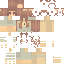### a l m o s t - t h e r e

Posted on: Apr 08, 2022
270
127
3
- + ❀ + - s h o w m o r e - + ❀ + -

good day darlings! <3
I'm hoping you are having a wonderful day <3

Here's the video
https://youtu.be/p1DYwZ6_eLg

=*.=*.=*.=*.=*.=*.=*.=*.=*.=*.=*.=*.=*.=
Current followers 489
Show Less

### a l m o s t - t h e r e

Posted on: Apr 08, 2022
270
127
3
- + ❀ + - s h o w m o r e - + ❀ + -

good day darlings! <3
I'm hoping you are having a wonderful day <3

Here's the video
https://youtu.be/p1DYwZ6_eLg

=*.=*.=*.=*.=*.=*.=*.=*.=*.=*.=*.=*.=*.=
Current followers 489